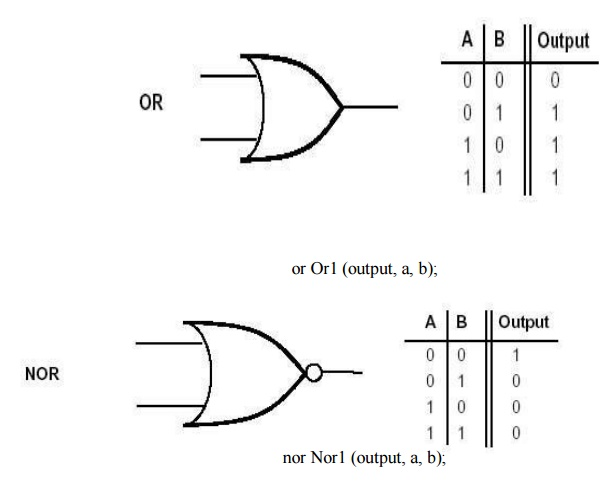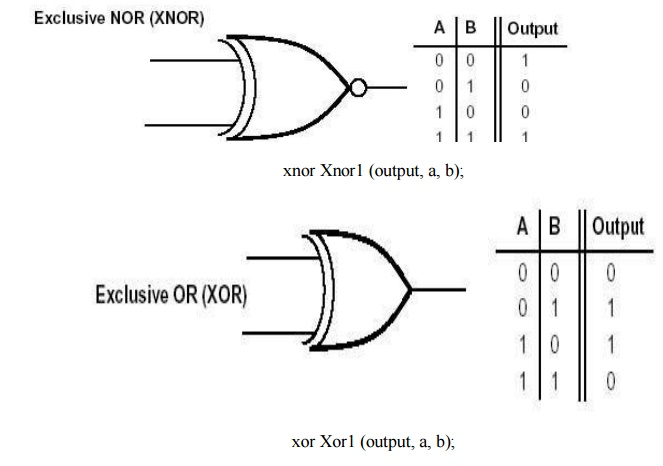Home | | VLSI Design | Gate Primitives

# Gate Primitives

Verilog defines some basic logic gates as part of the language. Gate primitives used in verilog codes are NOT, AND, OR, NAND, NOR, XOR, XNOR gate.

GATE PRIMITIVES

Verilog defines some basic logic gates as part of the language. Gate primitives used in verilog codes are NOT, AND, OR, NAND, NOR, XOR, XNOR gate. Verilog includes predefined modules that implement basic logic gates. These gates allow a circuit’s structure to be described using gate instantiation statements of the form:

gate_name [instance_name] (output_port, input_port {, input_port});

Here gate_name specifies the gate type and instance_name is an identifier, also it is optional.

Each gate may have different number of ports, the output port listed first, followed by a variable number of input ports.Structural specification of a full adder:

input a, b, cin; output sum, carry; wire p, q, r;

and And1 (p, a, b); and And2 (q, a, cin); and And3 (r, b, cin); or Or1 (carry, p, q, r);

xor Xor1 (sum, a, b, cin);

end module

input a, b, cin; output sum, carry; wire p, q, r;

and (p, a, b); and (q, a, cin); and (r, b, cin);

or (carry, p, q, r);

xor (sum, a, b, cin);

end module

Study Material, Lecturing Notes, Assignment, Reference, Wiki description explanation, brief detail
VLSI Design : Specification Using Verilog HDL : Gate Primitives |